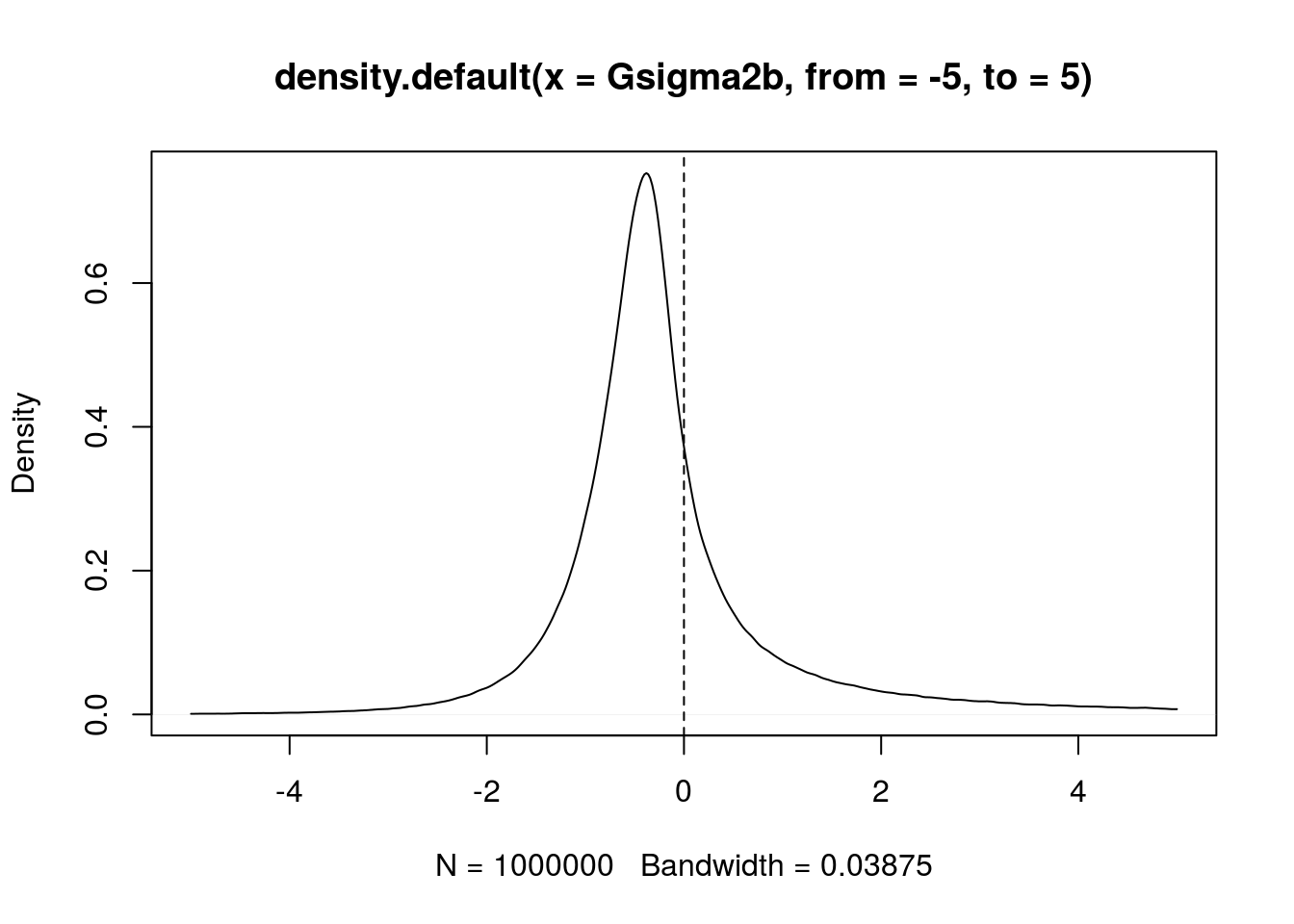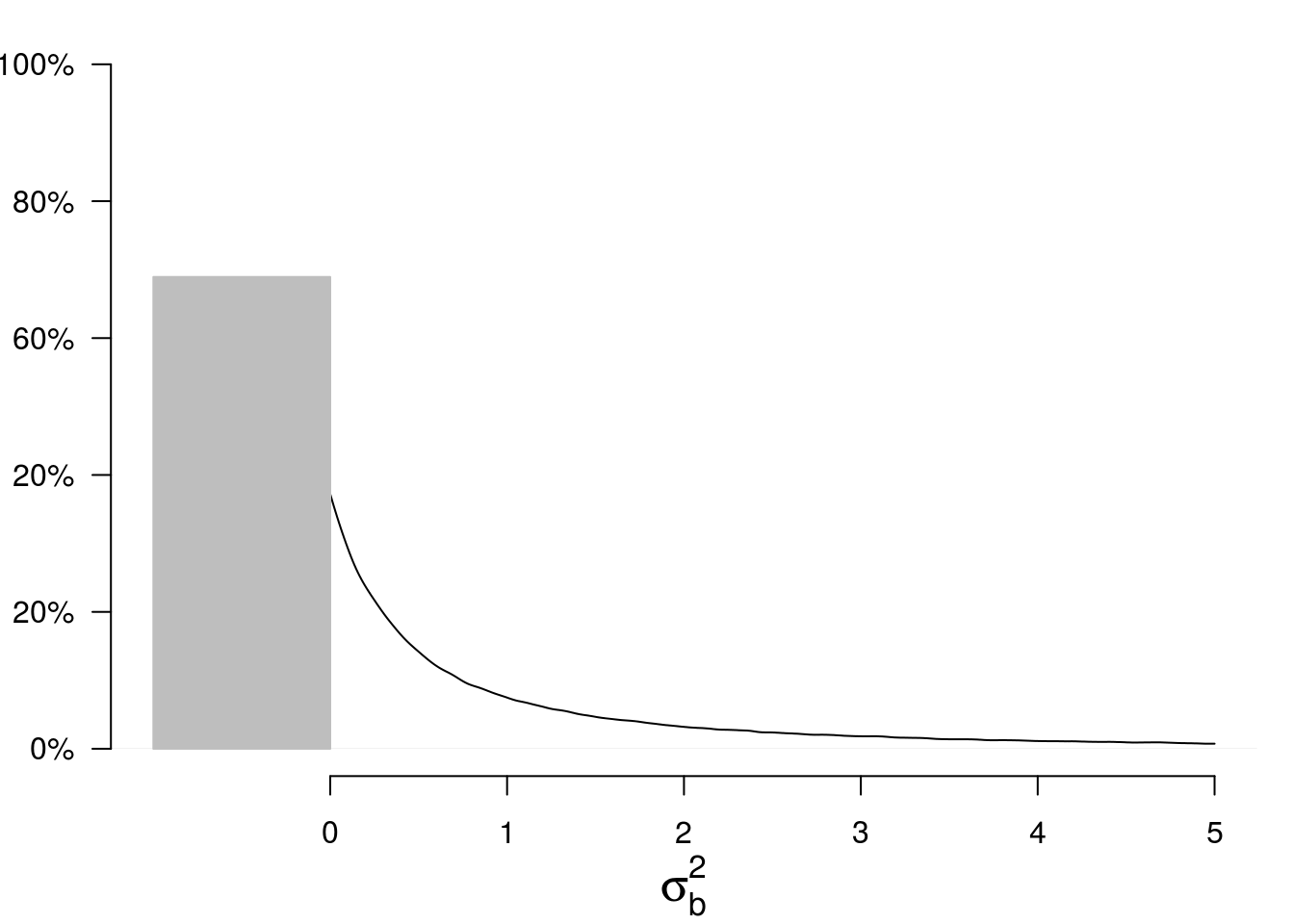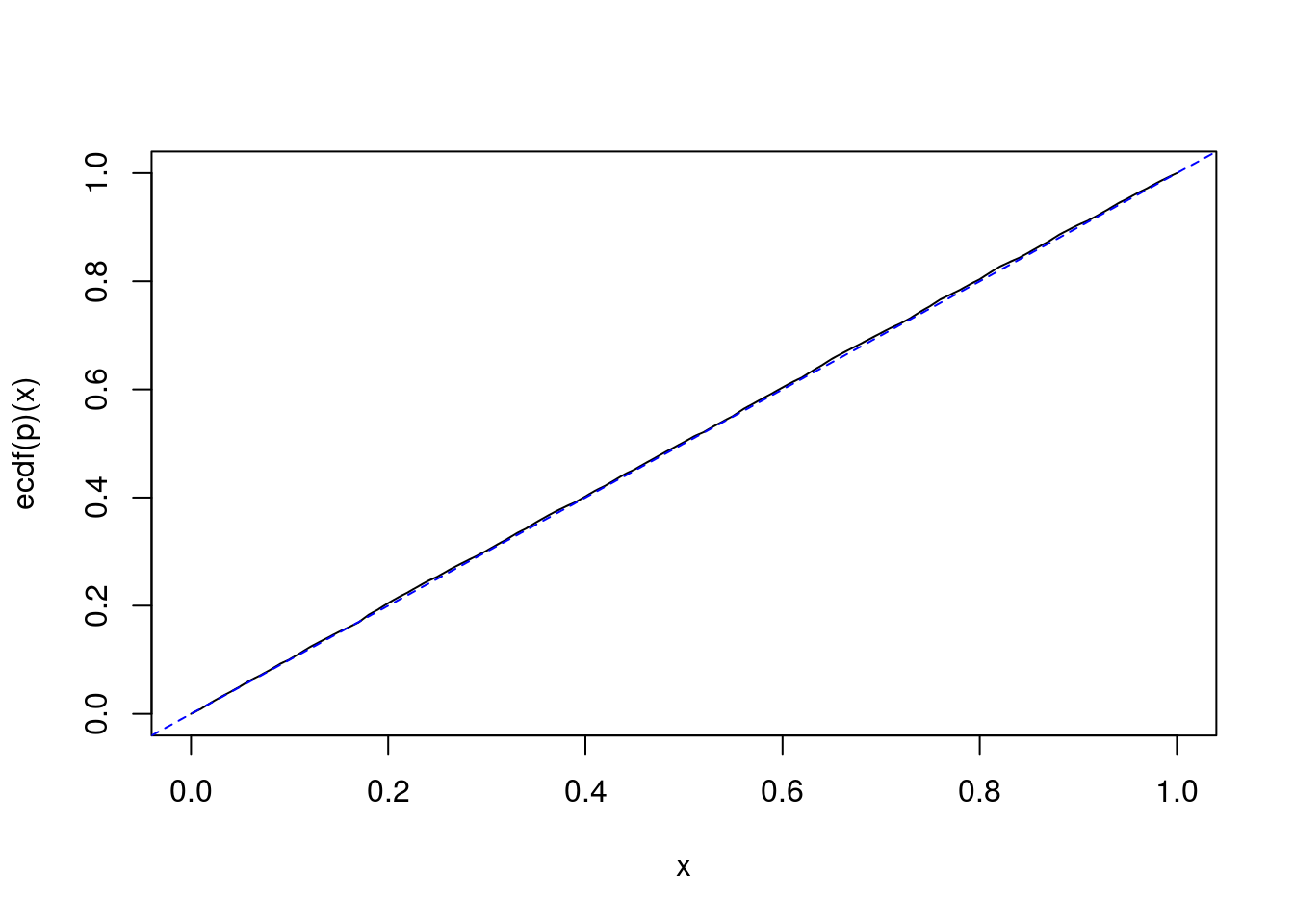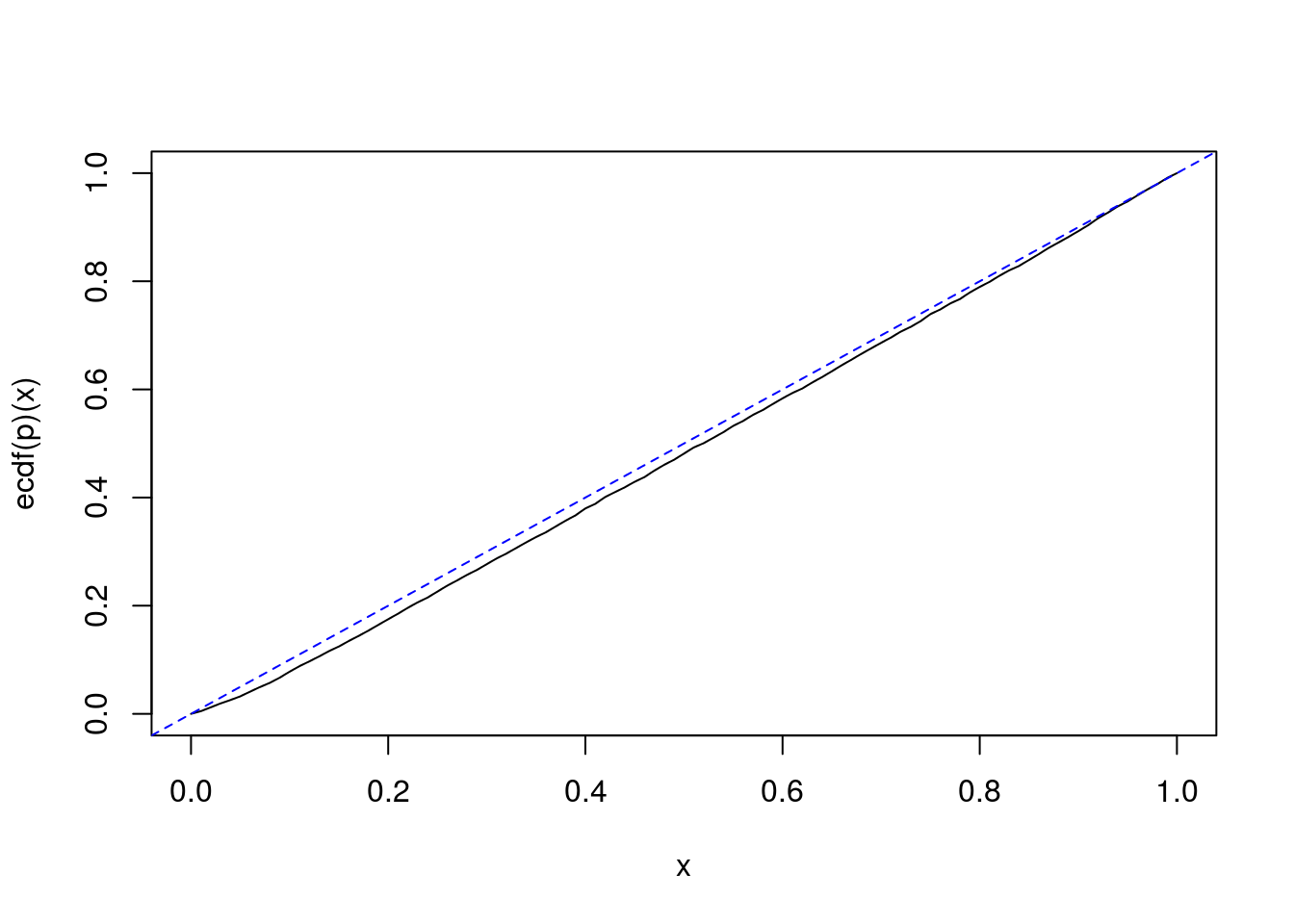1. Testing the nullity of the between variance

2017-04-02
Source

Recall that we consider the balanced one-way random effect ANOVA model. We use the index $i\in\{1,\ldots,I\}$ for the group index and the index $j\in\{1,\ldots,J\}$ for the observation index within a group.

The function SimData below simulates from this model.

library(data.table)
SimData <- function(I, J, mu, sigmab, sigmaw){
group <- gl(I, J, labels=LETTERS[1:I])
DT <- data.table(group = group,
y = mu + rep(rnorm(I, sd=sigmab), each=J) + rnorm(I*J, sd=sigmaw),
key = "group")
return(DT)
}
( DT <- SimData(I=2, J=3, mu=0, sigmab=1, sigmaw=2) )
##    group          y
## 1:     A -0.3734198
## 2:     A  1.6443825
## 3:     A  0.8613981
## 4:     B -6.6141322
## 5:     B -0.3080895
## 6:     B -3.6330317

The summaryStats function below calculates the three summary statistics $\bar y$, ${\mathrm{ss}}_b$ and ${\mathrm{ss}}_w$.

summaryStats <- function(DT){
DT[, :=(means = rep(mean(y), each=.N)), by=group]
ssw <- DT[, { squares = (y-means)^2
.(ssw = sum(squares))}]$ssw ybar <- mean(DT$y)
DT[, :=(Mean = ybar)]
ssb <- DT[, { squares = (means-Mean)^2
.(ssb = sum(squares))}]\$ssb
return(c(ybar=ybar, ssb=ssb, ssw=ssw))
}
summaryStats(DT)
##      ybar       ssb       ssw
## -1.403815 26.829259 21.972580

The distribution of the generalized pivotal quantity $G_{\sigma^2_b}$ (see previous article) can be seen as a “posterior distribution” of $\sigma^2_b$:

set.seed(666)
I <- 3L; J <- 4L
mu <- 0; sigmab <- 0; sigmaw <- 2
#
n <- 1e6L
Z <- rnorm(n); U2b <- rchisq(n, I-1); U2w <- rchisq(n, I*(J-1))
#
sss <- summaryStats(SimData(I, J, mu, sigmab, sigmaw))
Gsigma2b <- 1/J*(sss["ssb"]/U2b - sss["ssw"]/U2w)
plot(density(Gsigma2b, from=-5, to=5))
abline(v=0, lty="dashed")However, the between variance $\sigma^2_b$ is a positive parameter. Therefore it makes sense to replace $G_{\sigma^2_b}$ with $\max\bigl\{0, G_{\sigma^2_b}\bigr\}$. Thus, our “posterior distribution” becomes a mixture of a Dirac mass at $0$ and a density on the positive numbers:

par(mar=c(4,3,1,1))
p <- mean(Gsigma2b<0)
plot(density(Gsigma2b, from=0, to=5), main=NA,
xlim=c(-1,5), ylim=c(0,1),
xlab=expression(sigma[b]^2), ylab=NA,
axes=FALSE, cex.lab=1.5)
polygon(x=c(0,0,-1,-1), y=c(0,p,p,0),
col="gray", border="gray")
axis(1, at=0:5)
axis(2, at=seq(0,1,by=.2), las = 2,  labels=c("0%","20%","20%","60%","80%","100%"))The mass at $0$ is the posterior probability that $\sigma^2_b = 0$. Let us call $p$ this probability.

It is quite interesting to observe the following fact. If $\sigma^2_b = 0$, then simulations show that $p$ seemingly follows a uniform distribution on $[0,1]$:

I <- 2L; J <- 3L
mu <- 0; sigmab <- 0; sigmaw <- 2
#
n <- 1e6L
U2b <- rchisq(n, I-1); U2w <- rchisq(n, I*(J-1))
#
nsims <- 10000L
SIMS <- t(vapply(1:nsims, function(i){
summaryStats(SimData(I, J, mu, sigmab, sigmaw))
},
FUN.VALUE=numeric(3)))
p <- numeric(nsims)
for(i in 1:nsims){
ssb <- SIMS[i,"ssb"]; ssw <- SIMS[i,"ssw"]
Gsigma2b <- 1/J*(ssb/U2b - ssw/U2w)
p[i] <- mean(Gsigma2b<0)
}
curve(ecdf(p)(x))
abline(0, 1, lty="dashed", col="blue")In fact, it exactly follows a uniform distribution. We will easily prove it.

Therefore, it provides a perfect $p$-value for the null hypothesis $H_0\colon\{\sigma^2_b=0\}$, that is, rejecting $H_0$ when $p < \alpha$ provides a test with significance level $\alpha$.

Proof

Assuming $\sigma^2_b=0$, \begin{align*} \Pr(G_{\sigma^2_b} < 0) & = \Pr\left(\frac{U^2_w}{U^2_b} < \frac{{\mathrm{ss}}_w}{{\mathrm{ss}}_b} \right) \\ & = F\left(\frac{{\mathrm{ss}}_w}{{\mathrm{ss}}_b} \right) \end{align*} where $F$ is the cdf of $\frac{U^2_w}{U^2_b}$.

The result follows from the fact that $\frac{{\mathrm{SS}}_w}{{\mathrm{SS}}_b}$ has the same distribution as $\frac{U^2_w}{U^2_b}$ when $\sigma^2_b=0$, and from the well-known fact that $G(X)$ follows the uniform distribution on $[0,1]$ whenever $X$ is a continuous random variable and $G$ is its cdf.

One-sided confidence intervals

Simulations also show that the above property almost holds for the null hypothesis $H_0\colon\{\sigma^2_b=\theta_0\}$ for any $\theta_0$, versus the one-sided alternative hypothesis $H_1\colon\{\sigma^2_b>\theta_0\}$ or $H_1\colon\{\sigma^2_b<\theta_0\}$. That would mean that the one-sided generalized confidence intervals about $\sigma^2_b$ are almost exact confidence intervals.

sigmab <- 2
SIMS <- t(vapply(1:nsims, function(i){
summaryStats(SimData(I, J, mu, sigmab, sigmaw))
},
FUN.VALUE=numeric(3)))
p <- test <- numeric(nsims)
for(i in 1:nsims){
ssb <- SIMS[i,"ssb"]; ssw <- SIMS[i,"ssw"]
Gsigma2b <- 1/J*(ssb/U2b - ssw/U2w)
p[i] <- mean(Gsigma2b<sigmab^2)
test[i] <- sigmab^2 < quantile(Gsigma2b, 0.95)
}
curve(ecdf(p)(x))
abline(0, 1, lty="dashed", col="blue")# coverage
mean(test)
##  0.9476